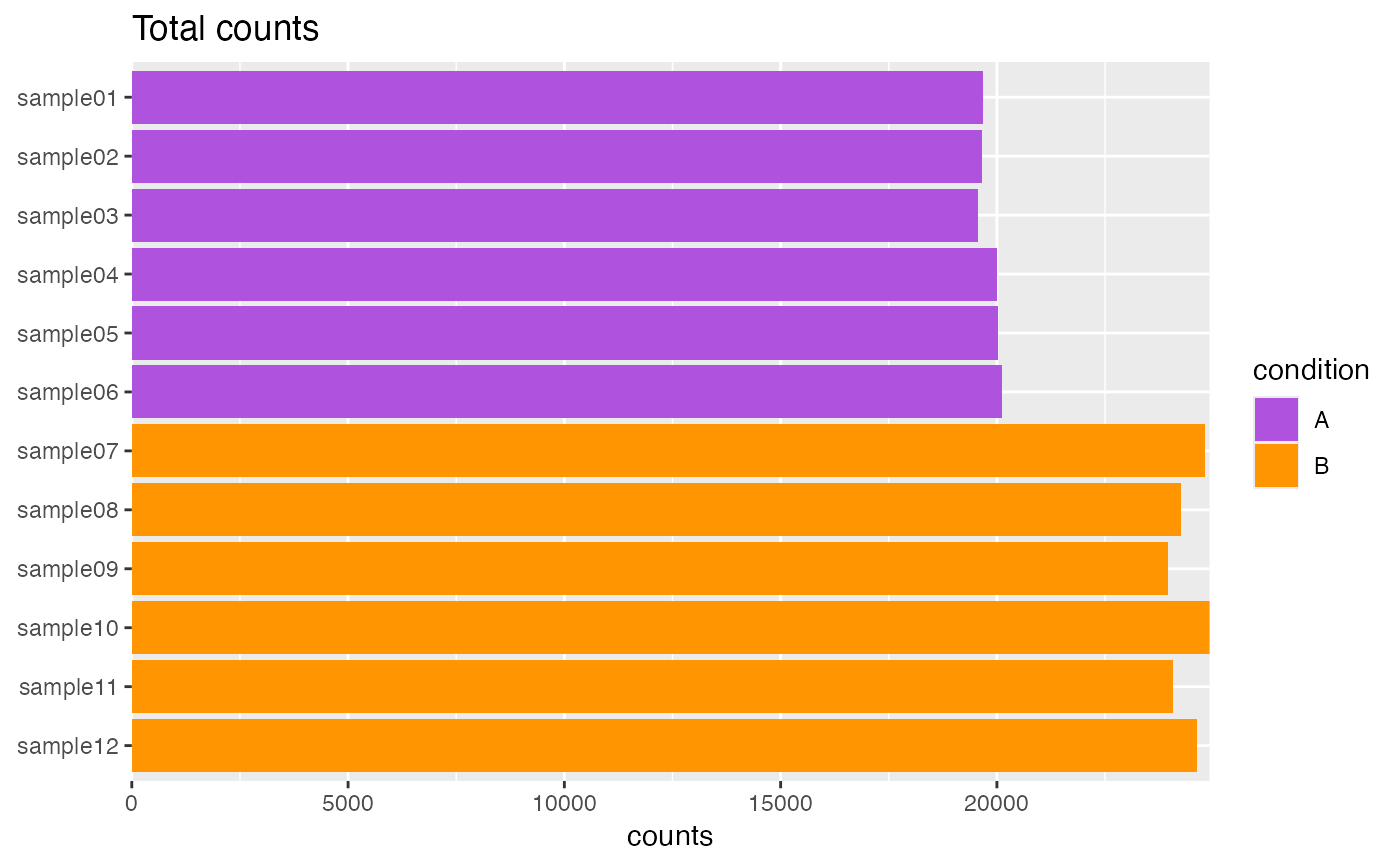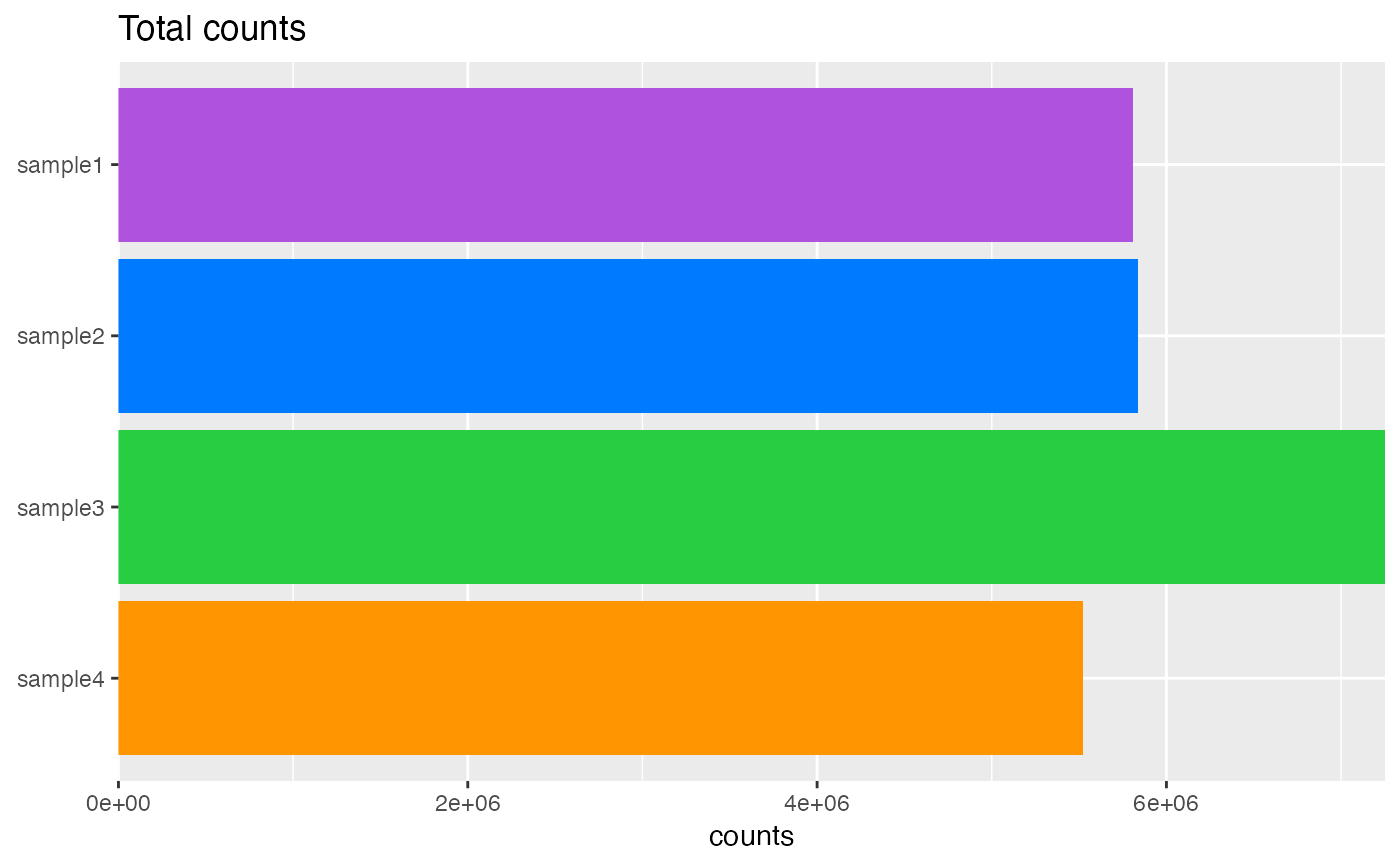## Usage

plotTotalCounts(object, ...)

# S4 method for SingleCellExperiment
plotTotalCounts(object, ...)

# S4 method for SummarizedExperiment
plotTotalCounts(
object,
assay = 1L,
interestingGroups = NULL,
perMillion = FALSE,
labels = list(title = "Total counts", subtitle = NULL, x = NULL, y = "counts"),
flip = getOption(x = "acid.flip", default = TRUE)
)

## Arguments

object

Object.

...

assay

vector(1). Assay name or index position.

interestingGroups

character. Groups of interest to use for visualization. Corresponds to factors describing the columns of the object.

perMillion

logical(1). Display as counts per million.

labels

list. ggplot2 labels. See ggplot2::labs() for details.

flip

logical(1). Flip x and y axes. Recommended for plots containing many samples.

## Value

ggplot.

## Functions

• plotTotalCounts(SingleCellExperiment): Applies aggregateCellsToSamples() calculation to summarize at sample level prior to plotting.
Passes ... to SummarizedExperiment method.

## Note

Updated 2022-03-04.

## Examples

data(
RangedSummarizedExperiment,
SingleCellExperiment_splatter,
package = "AcidTest"
)

## SummarizedExperiment ====
object <- RangedSummarizedExperiment
plotTotalCounts(object)## SingleCellExperiment ====
object <- SingleCellExperiment_splatter
plotTotalCounts(object)